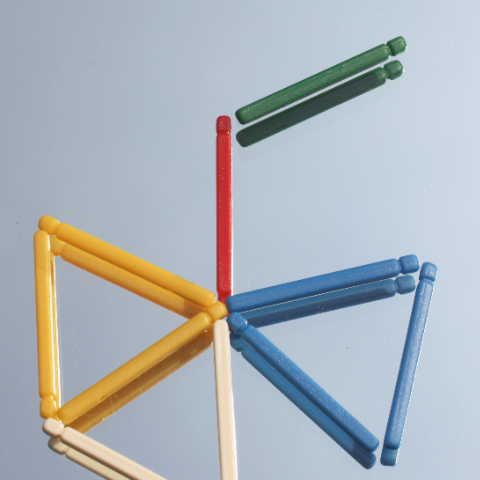# Philosophy of Mathematics Seminar (Week 8, TT18)The study of hierarchies in mathematics has been a very active field of research since the 1940s. Hierarchies are usually taken to classify objects (or collections thereof) according to a measure of complexity along some dimension, such that the level at which a given object appears in the hierarchy measures the degree of difficulty of constructing the object in question, or equivalently, the difficulty of verifying its existence. Theories considered mathematically ‘natural’ often occupy special places in these hierarchies, and can sometimes be put into interesting correspondences with foundational or philosophical approaches to mathematics, according to the strength of their existential assumptions.

In this talk, I will propose a different, complementary view of the lessons we can draw from the study of mathematical hierarchies. According to this view, hierarchies measure the relative distance from the axiomatic assumptions that we make on the basis of our pre-theoretical understanding of a certain domain of mathematical objects, by means of countable or uncountable iterations of inference patterns that we recognise as correct. In outlining this view, I will consider how the study of hierarchies developed from the new formal analyses of concepts such as ’computation’, ’consistency’, ’interpretation’, and ’model’ that emerged in the early 20th century, and I will suggest that a deeper understanding of the historical development of mathematical hierarchies can help illuminate their significance

Philosophy of Mathematics Seminar Convenors: Dr James Stud, Dr Daniel Isaacson, and Prof Volker Halbach